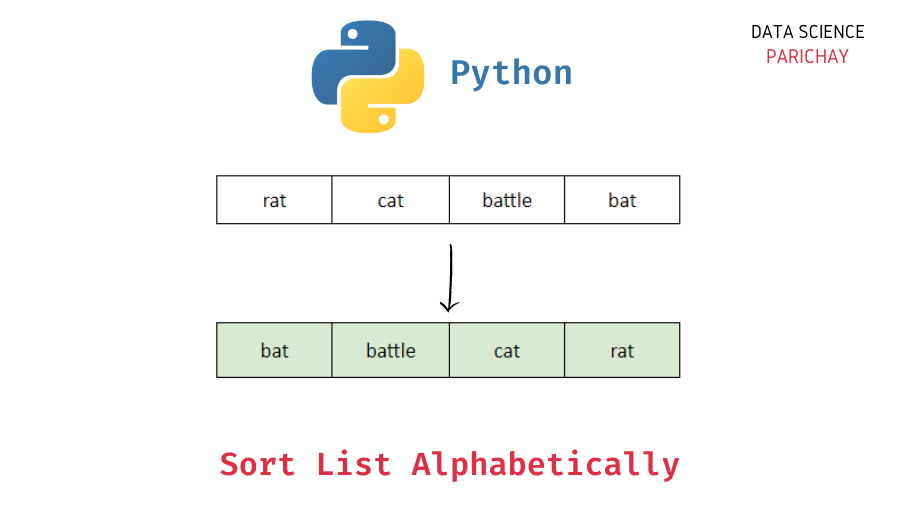# Python – Sort List of Strings Alphabetically

In this tutorial, we will look at how to sort a list of strings in alphabetical order in Python with the help of some examples.

You can use the Python built-in `sorted()` function to sort a list. You can also use this function to sort other iterables like tuple, string, etc. The following is its syntax:

```# sort a list
new_ls = sorted(ls)```

It returns a new list with the elements in sorted order and doesn’t modify the original list. By default, it sorts the list in ascending order but you can also sort the list in descending order by passing `reverse=True`.

In Python, comparing strings to determine the order works by comparing the Unicode of the first characters in both the strings. If the first character is the same, it compares the second character in each string, and so on. For example –

`"big" < "bin"`

Output:

`True`

Let’s see the usage of the `sorted()` function to sort strings in a list in alphabetical order.

```# create a list
ls = ['bat', 'pat', 'cat', 'mat', 'rat', 'battle']
# sort the list
sorted_ls = sorted(ls)
# display the list
print(sorted_ls)```

Output:

📚 Data Science Programs By Skill Level

Introductory

Intermediate ⭐⭐⭐

🔎 Find Data Science Programs 👨‍💻 111,889 already enrolled

Disclaimer: Data Science Parichay is reader supported. When you purchase a course through a link on this site, we may earn a small commission at no additional cost to you. Earned commissions help support this website and its team of writers.

`['bat', 'battle', 'cat', 'mat', 'pat', 'rat']`

You can see that returned list contains strings in alphabetical order. Here the words “battle” and “bat” start with the same letters but “battle” is considered larger than “bat” due to its length.

You can also specify the order to be reversed.

```# create a list
ls = ['bat', 'pat', 'cat', 'mat', 'rat', 'battle']
# sort the list
sorted_ls = sorted(ls, reverse=True)
# display the list
print(sorted_ls)```

Output:

`['rat', 'pat', 'mat', 'cat', 'battle', 'bat']`

The words are now in the reverse order.

Note that, uppercase characters are considered “smaller” than lowercase characters in string comparison.

```# create a list
ls = ['bat', 'pat', 'cat', 'Mat', 'rat', 'battle']
# sort the list
sorted_ls = sorted(ls)
# display the list
print(sorted_ls)```

Output:

`['Mat', 'bat', 'battle', 'cat', 'pat', 'rat']`

You can see that even though alphabetically “Mat” comes after “bat” it is sorted in before it because it starts with an uppercase letter. This is because uppercase characters have lower Unicode values compared to lowercase characters (remember that we compare the Unicode values starting from the first character to determine the order).

If you want to sort them alphabetically irrespective of the case, you can use the `key` parameter which is used to specify the function to be applied to each element. The result of this function is used to compare the values when sorting.

```# create a list
ls = ['bat', 'pat', 'cat', 'Mat', 'rat', 'battle']
# sort the list
sorted_ls = sorted(ls, key=str.lower)
# display the list
print(sorted_ls)```

Output:

`['bat', 'battle', 'cat', 'Mat', 'pat', 'rat']`

You can see that now the strings are sorted alphabetically irrespective of their case.

You can pass your own custom criteria to the `key` argument to sort the values. For example, if you want to sort the strings in a list based just on their length –

```# create a list
ls = ['bat', 'pat', 'battle', 'cat', 'Mat', 'rat']
# sort the list
sorted_ls = sorted(ls, key=len)
# display the list
print(sorted_ls)```

Output:

`['bat', 'pat', 'cat', 'Mat', 'rat', 'battle']`

The strings in the list are sorted based on their length. For strings with equal lengths, we see that they are present in the order they occur in the original list.

Alternatively, you can use the Python list `sort()` function. It sorts the list in place and doesn’t return any value. That is, it directly modifies the original list.

Note that the `sort()` function works only on lists. You cannot use it on other iterables like tuple, string, etc. Let’s look at an example of using it to alphabetically sort a list of strings.

```# create a list
ls = ['bat', 'pat', 'cat', 'mat', 'rat', 'battle']
# sort the list
ls.sort()
# display the list
print(ls)```

Output:

`['bat', 'battle', 'cat', 'mat', 'pat', 'rat']`

The original list is now in alphabetical order.

•